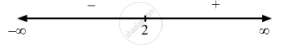Share

F(X) = X 2 + 2 X , X > 0 . - CBSE (Science) Class 12 - Mathematics

Question

f(x) =$\frac{x}{2} + \frac{2}{x} , x > 0$ .

Solution

$\text { Given }: \hspace{0.167em} f\left( x \right) = \frac{x}{2} + \frac{2}{x}$

$\Rightarrow f'\left( x \right) = \frac{1}{2} - \frac{2}{x^2}$

$\text { For the local maxima or minima, we must have }$

$f'\left( x \right) = 0$

$\Rightarrow \frac{1}{2} - \frac{2}{x^2} = 0$

$\Rightarrow \frac{1}{2} = \frac{2}{x^2}$

$\Rightarrow x^2 = \pm 2$Since x > 0,  f '(x) changes from negative to positive when x increases through 2. So, x = 2 is a point of local minima.

The local minimum value of  f (x) at x = 2 is given by $\frac{2}{2} + \frac{2}{2} = 2$

Is there an error in this question or solution?

Video TutorialsVIEW ALL 

Solution F(X) = X 2 + 2 X , X > 0 . Concept: Graph of Maxima and Minima.
S